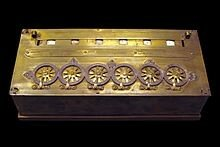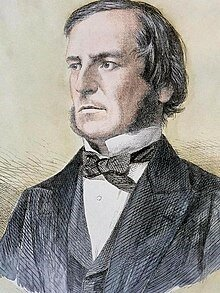• # Blaise Pascal invented the first mechanical calculator, the Pascaline.The calculator could add and subtract and inspired Gottfried Leibniz, whom many consider being the first computer scientist, to build the first mechanical calculator that could perform all four basic arithmetic functions in 1685.
• # George Boole publishes "Mathematical Analysis of Logic."1847 George Boole, the inventor of Boolean algebra publishes his work “Mathematical Analysis of Logic.” Used to this day, Boolean algebra is fundamental to the world of electronics and computer science as Boolean Logic represents mathematics that can only have True, False, or 0,1 as outcomes.
• # The ENIAC computer is built.The first programmable electronic computer was built. Called the ENIAC, it was built by John Mauchly and J. Presper Eckert. The ENIAC at the time was one of the largest computers built and was dubbed the “Giant Brain” by the press. It was one thousand times faster than computing machines prior to it. Although much excitement was expressed for its potential, the machine used over 17,000 vacuum tubes. Many scientists recognized the limitations of space, cost, and construction of such computers.
• # The first point contact transistor is invented by Bell LabsIn 1947, John Bardeen, and Walter Brattain at Bell Labs invented the first point-contact transistor thus revolutionizing computers forever. Sparking a new age in computing, that will replace the vacuum tube era of computing, the transistor solves many of the problems that the vacuum tubes presented. Bell Labs would further develop the transistor by inventing the first MOSFET transistor which is still used to this day.
• # Moore's Law popularizedA former member of Bell Labs, Gordon Moore hypothesized that the number of transistors on a chip would double every two years. Known in the industry as Moore’s law, the law unintentionally set the pace of the industry instead of simply predicting it. Used to this day, many predict that the industry is reaching the end of Moore's Law as transistors and semiconductor chips push the laws of physics.